##### Least Squares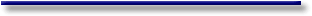# Ordinary Least Squares for Simple Regression

#### II.I.2 Ordinary Least Squares for Simple Regression

Assume the following relationship should be investigated by means of simple linear regression

the influence of the interest rate on the demand of new private cars.

If the researcher has two equally ranged time series, R for interest rate) and C (for the demand of cars) the following mathematical model could be considered: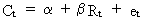(II.I.2-1)

This is a linear relationship between C and R where ß is equal to the sensitivity of C to R, alpha is equal to a constant term, and et is equal to the error term.

Of course one would expect beta to have a negative sign since high interest rates would de-motivate potential car buyers, while low interest rates would give them an incentive to buy more new cars (due to cheaper loans).

It is quite important to understand that in classic econometrics the specification of the relationship to be considered such as eq. (II.I.2-1) is based on economic theory (and common sense).

If the necessary data (e.g. demand for new cars and interest rates) are at hand, and if the researcher has a good economic specification, all he needs is a mathematical technique to compute the relationship.

Quite often, econometrics is therefore defined as the intersection of three sets: economic theory, economic statistics (data), and mathematical techniques.

Before we tackle the problem of computing a and ß it must be understood that any technique of calculating the parameters would generate some statistic error. This error will occur because of the fact that the researcher only has two observed time series which should be seen as a set of samples, drawn from a population of all possible interest rates and all possible car sales volumes (at any given moment in time). This is the reason why we will never be able to calculate the true value of alpha and beta. However, it is possible to estimate the values of alpha and beta as good as possible (using the least squares criterion).

We rewrite the previous model by replacing alpha with the estimated value of alpha and beta with the estimated value of beta: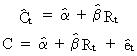(II.I.2-2)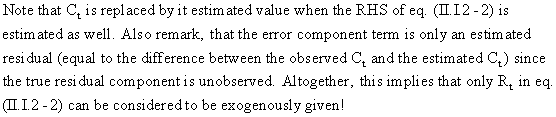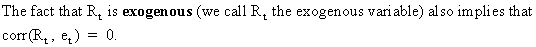Now we will derive the formulae for the estimated alpha and beta for a simple linear model (c.q. the estimator)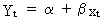(II.I.2-3)

(assuming a zero error component) and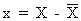(II.I.2-4)

Using the first order condition it is possible to find a solution for both parameters that minimize the SSR.

First we calculate the partial derivative (of the SSR) with respect to alpha and equate it to zero to find the optimum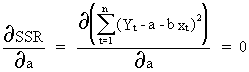(II.I.2-5)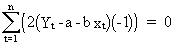(II.I.2-6)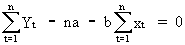(II.I.2-7)(II.I.2-8)

where in equation (II.I.2-7)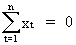(II.I.2-9)

since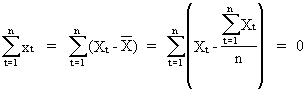(II.I.2-10)

Secondly, we calculate the partial derivative of the SSR with respect to beta and equate it to zero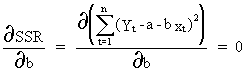(II.I.2-11)(II.I.2-12)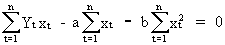(II.I.2-13)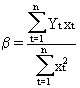(II.I.2-14)

In practice eq. (II.I.2-8) and eq. (II.I.2-14) are used to estimate the values of alpha and beta by the so-called OLS technique. Remember that in case of parameter estimation we replace the symbols alpha and beta by their estimated values (these are indicated by a hat above the respective symbol).

In order to ensure that (II.I.2-5) and (II.I.2-11)have been minimized (and not maximized) the second order partial derivatives should be calculated. These derivatives will have a positive sign (verify this). This proves that the two optima we have found, do correspond with the minima of the SSR.

If a forecast of Yt has to be made, one may use the following expression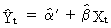(II.I.2-15)

where the estimated value of a from (II.I.2-8) has to be replaced by(II.I.2-16)

because we previously transformed the exogenous variable according to eq. (II.I.2-4).

In this discussion of OLS we implicitly made some crucial assumptions. If these assumptions are not satisfied, OLS is not applicable.

##### Example of an interpolation forecast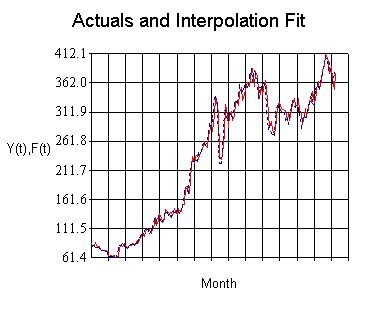© 2000-2022 All rights reserved. All Photographs (jpg files) are the property of Corel Corporation, Microsoft and their licensors. We acquired a non-transferable license to use these pictures in this website.
The free use of the scientific content in this website is granted for non commercial use only. In any case, the source (url) should always be clearly displayed. Under no circumstances are you allowed to reproduce, copy or redistribute the design, layout, or any content of this website (for commercial use) including any materials contained herein without the express written permission.

Information provided on this web site is provided "AS IS" without warranty of any kind, either express or implied, including, without limitation, warranties of merchantability, fitness for a particular purpose, and noninfringement. We use reasonable efforts to include accurate and timely information and periodically updates the information without notice. However, we make no warranties or representations as to the accuracy or completeness of such information, and it assumes no liability or responsibility for errors or omissions in the content of this web site. Your use of this web site is AT YOUR OWN RISK. Under no circumstances and under no legal theory shall we be liable to you or any other person for any direct, indirect, special, incidental, exemplary, or consequential damages arising from your access to, or use of, this web site.

Contributions and Scientific Research: Prof. Dr. E. Borghers, Prof. Dr. P. Wessa
Please, cite this website when used in publications: Xycoon (or Authors), Statistics - Econometrics - Forecasting (Title), Office for Research Development and Education (Publisher), http://www.xycoon.com/ (URL), (access or printout date).